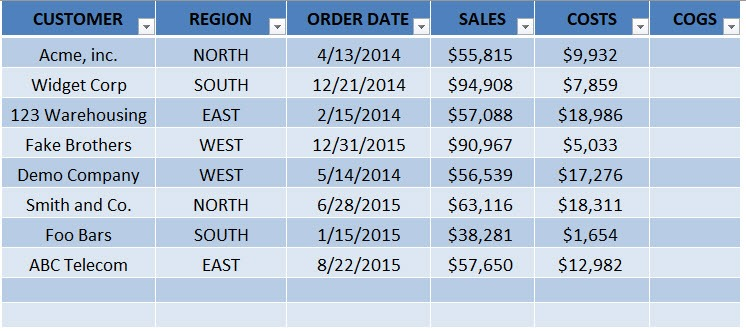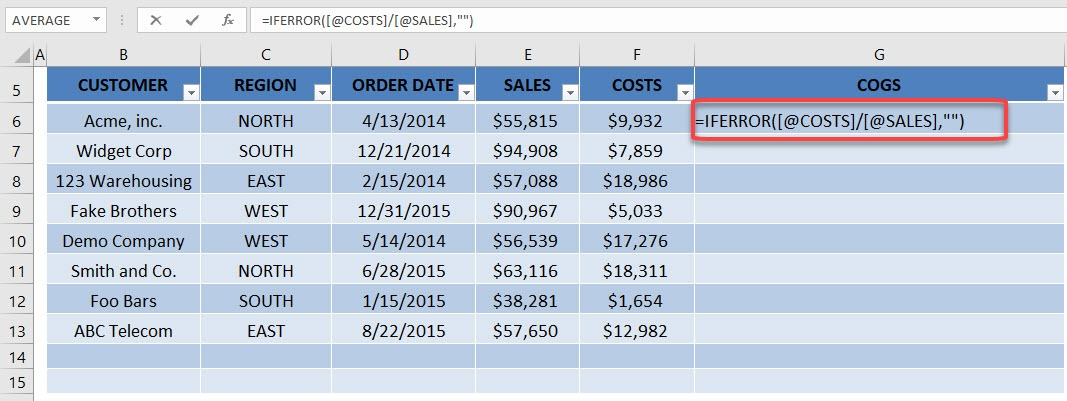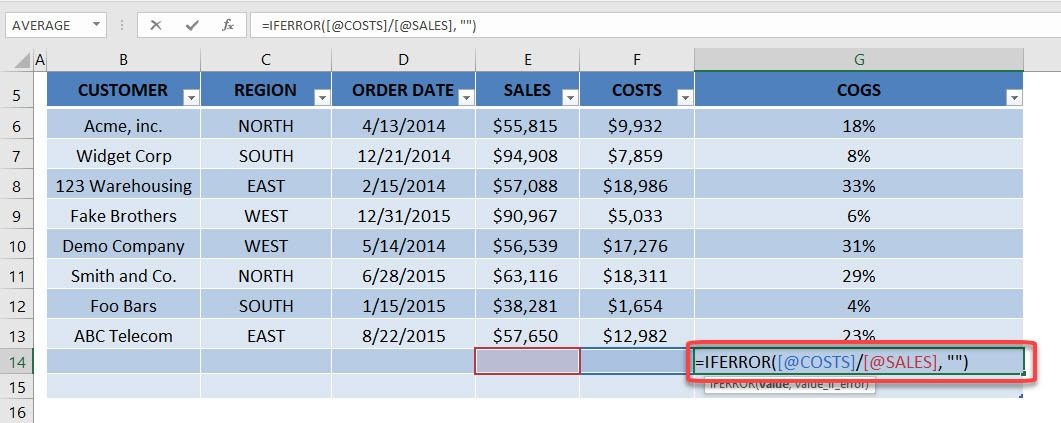One of the advantages of using an Excel Table is the ability to autofill a formula all the way down your data without having to copy and paste.

When you write a formula anywhere in your Excel Table, it will automatically fill down and up within that column.

As you add extra rows to your Excel Table, the formula fills in to the extra rows added, so you do not need to copy and paste.

How efficient is that!

This is our starting data and has been converted into an Excel Table. Our goal is to compute the percentage of costs in our sales amount then put it in the blank column.STEP 1: Type in this formula to get the percentage of costs in sales. We are using IFERROR to account for blank values.

## =IFERROR([@COSTS]/[@SALES], “”)STEP 2: Pick on any random row and notice that the formula has been applied to that as well. This is the power of using Excel Tables and your formula has been applied to the entire column! No more copy pasting!